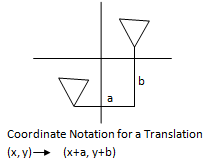Email us to get an instant 20% discount on highly effective K-12 Math & English kwizNET Programs!

#### Online Quiz (WorksheetABCD)

Questions Per Quiz = 2 4 6 8 10

### Geometry7.4 Translation

 Translation Mapping: A mapping of a plane in which each point of the plane moves in the same direction by the segments, all congruent to a given segment (equal distance) is called a translation. Translation moves every point of a figure the same distance in the same direction.Examples: Find the coordinates of the image of (2,7), under the translation defined by (x,y) ® (x+2, y+1). T(x,y) ® (x+2, y+1). T(2,7) ® (2+2, 7+1). The coordinates of the image is (4,8) A quadrilateral has vertices A(-4,3), B(-2,4), C(-1,1) and D(-3,1). Find the coordinates of the image after translation (x,y) ® (x+5,y-2). (x,y) ® (x+2, y+1). A(-4,3) ® (1, 1) B(-2,4) ® (3, 2) C(-1,1) ® (4, -1) D(-3,1) ® (2, -1) Directions: Choose the correct answer. Also write at least 5 examples of your own.
 Q 1: Point on image: (6, -9); translation: (x,y) --> (x-7, y-4) Find the corresponding point on the original figure. (-1,-13)(13, -5)(-4, 10) Q 2: Find the coordinates of the image of (4, 9), under the translation defined by (x,y) ® (x+4, y-2).(8, 7)(7, 8)(6, 8) Q 3: Find the coordinates of the image of (5, 6), under the translation defined by (x,y) ® (x+2, y-1).none(5, 7)(7, 5) Q 4: Find the coordinates of the image of (2, 8), under the translation defined by (x,y) ® (x, y-5).(3, -4)(4, 3)(3, 4) Q 5: Find the coordinates of the image of (3, 4), under the translation defined by (x,y) ® (x+2, y-1).(5,3)(3,5)(5,5) Q 6: If (0,3) translates to (1,2), then (2,5) translates to _______(2, 5)(4, 3)(3, 4) Question 7: This question is available to subscribers only! Question 8: This question is available to subscribers only!